Data-driven 4IR skills development

# Angles

## Angles

Angles are used in daily life.  Engineers and architects use angles for designs, roads, buildings and sporting facilities.  Athletes use angles to enhance their performance.  Carpenters use angles to make chairs, tables and sofas.  Artists use their knowledge of angles to sketch portraits and paintings.

If two lines meet (or intersect) at a point, then an angle is formed.  The point of intersection of the lines is called the vertex.

An angle is shown below.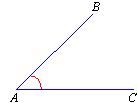Lines AB and AC meet at the point A to form an angle.  The point A is the vertex of the angle, and the lines that meet to make the angle are called the arms of the angle.

### Naming Angles### Size of an Angle

The amount of turn from one arm of the angle to the other is said to be the size of an angle.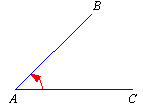The size of an angle is measured in degrees; and the symbol used to represent degree is º.  There are 360º in a full turn (or circle).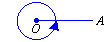###### Note:

degree is defined such that the angle of one full turn (or circle) is 360 degrees.

### Measuring Angles

protractor is used to measure angles.  In this section, we will consider the use of a protractor that has the shape of a semi-circle and two scales marked from 0º to 180º.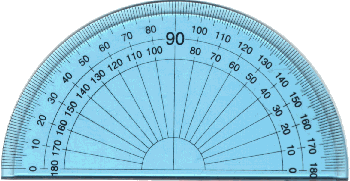The two scales make it easy for us to measure angles facing different ways.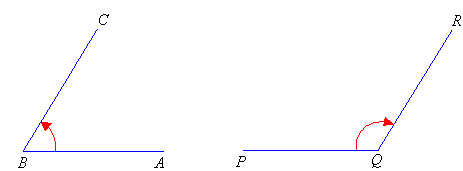To measure the size of angle ABC, place the protractor over the angle so that the centre of the protractor is directly over the angle’s vertex, B; and the base line of the protractor is along the arm, BA, of the angle.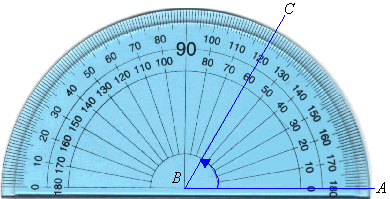We use the inner scale to measure the angle ABC, as the arm AB passes through the zero of the inner scale.  Following the inner scale around the protractor, we find that the other arm, BC, passes through the inner scale at 60º.  So, the size of angleABC is 60 degrees. We write this as follows: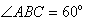To measure the size of angle PQR, place the protractor over the angle so that the centre of the protractor is directly over the angle’s vertex, Q; and the base line of the protractor is along the arm, PQ, of the angle.We use the outer scale to measure the angle PQR, as the arm PQ passes through the zero of the outer scale.  Following the outer scale around the protractor, we find that the other arm, QR, passes through the outer scale at 120º.  So, the size of angle PQR is 120 degrees.  We write this as follows: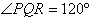### Types of Angles

Angles are classified according to their size.

An acute angle is greater than 0º and less than 90º.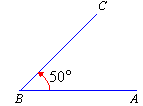right angle equals exactly 90º.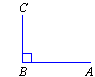Note that a right angle is marked on the diagram as a small square.

An obtuse angle is greater than 90º and less than 180º.A straight angle equals exactly 180º.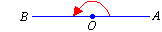reflex angle is greater than 180º and less than 360º.perigon (or a revolution) is an angle that equals exactly 360º.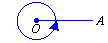### Measuring Reflex Angles

###### Recall that:

A protractor can be used to measure the size of an acute angle (between 0º and 90º) and an obtuse angle (between 90º and 180º).

Now, we will use a protractor to measure the reflex angle PQR.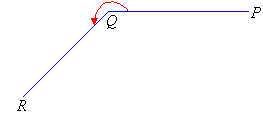To measure the reflex angle PQR, extend the arm PQ to A to form angle PQA which is a straight angle.  Then measure the size of the angle AQR and add 180º.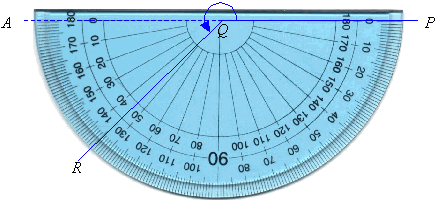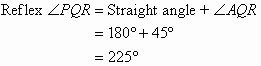https://mathsgee.com

Edzai Conilias Zvobwo is passionate about empowering Africans through mathematics, problem-solving techniques and media. As such, he founded MathsGee. Through this organisation, he has helped create an ecosystem for disseminating information, training, and supporting STEM education to all African people. A maths evangelist who teaches mathematical thinking as a life skill, Edzai’s quest has seen him being named the SABC Ambassador for STEM; he has been invited to address Fortune 500 C-suite executives at the Mobile 360 North America; was nominated to represent Southern Africa at the inaugural United Nations Youth Skills Day in New York; was invited to be a contributor to the World Bank Group Youth Summit in 2016; has won the 2014 SADC Protocol on Gender and Development award for his contribution to women’s empowerment in education; and has partnered with local and global firms in STEM interventions.

Comments are closed.

Loading...